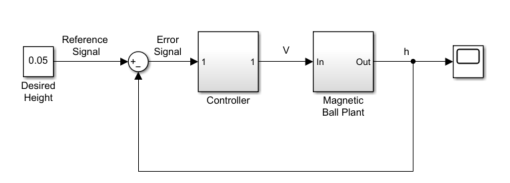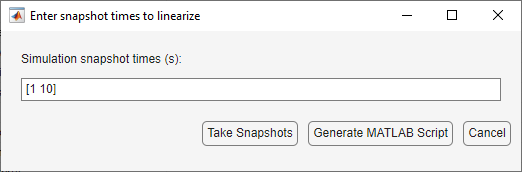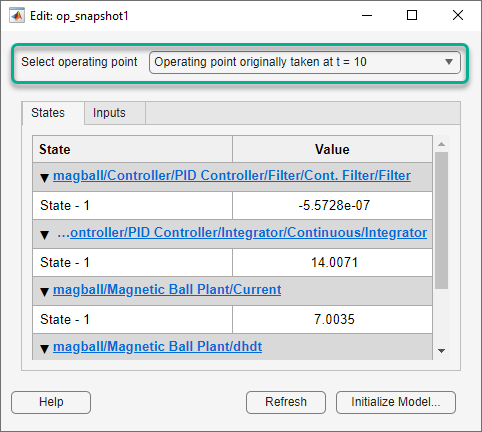Main Content

## Find Operating Points at Simulation Snapshots

You can find a steady-state operating point using a model simulation. The resulting operating point consists of the state values and model input levels at a specified simulation snapshot time.

To use simulation-based operating point computation, first configure your model initial conditions such that the model converges to an equilibrium point. You can then simulate your model and create operating points interactively using the Steady State Manager or Model Linearizer. You can also find snapshots programmatically at the MATLAB® command line using the `findop` function.

To find operating points using snapshots, the software simulates the model and creates an operating point at each simulation snapshot time. Each operating point contains the input and states values of the model at the corresponding snapshot time.

To verify that the operating point is at steady state, initialize your model with the operating point values, simulate the model, and check if key signals and states are at equilibrium. For more information on initializing your model with an operating point, see Simulate Simulink Model at Specific Operating Point.

Note

If your Simulink® model contains blocks with internal states, do not linearize the model at an operating point you compute from a simulation snapshot. Instead, try linearizing the model using a simulation snapshot or at an operating point found using trimming.

### Compute Operating Points at Simulation Snapshots Using Steady State Manager

You can find an operating point at specified simulation snapshot times using the Steady State Manager.

Open the Simulink model.

```sys = 'magball'; open_system(sys)```To open the Steady State Manager, in the Simulink model window, in the Apps gallery, click Steady State Manager.

To specify the simulation snapshot time, in the Steady State Manager, on the Steady State tab, click Snapshots.

Specify simulation ties at which to take snapshots. For this example, take snapshots `1` and `10` time units. In the Create Snapshot Operating Point dialog box, in the Simulation snapshot times field, enter ```[1 10]```.To take the snapshots, click.

An array of operating points, `op1`, appears in the Data Browser, in the Operating Points section. This array contains two operating points, one for each specified snapshot time.

The software also opens a corresponding op1 document where you can view the operating points.

To select which operating point to view, use the Select Operating Point drop-down list.### Compute Operating Points at Simulation Snapshots Using Model Linearizer

You can find an operating point at specified simulation snapshot times using the Model Linearizer.

Open the Simulink model.

```sys = 'magball'; open_system(sys)```To open the Model Linearizer, in the Simulink model window, in the Apps gallery, click Model Linearizer.

To specify the simulation snapshot time, in the Model Linearizer, on the Linear Analysis tab, in the Operating Point drop-down list, select ```Take Simulation Snapshot```.

Take simulation snapshots at `1` and `10` time units. In the Enter snapshot times to linearize dialog box, in the Simulation snapshot times field, enter `[1 10]`.To take the snapshots, click .

An array of operating points, `op_snapshot1`, appears in the Data Browser, in the Linear Analysis Workspace section. This array contains two operating points, one for each specified snapshot time.

To view the operating points, in the Linear Analysis Workspace, double-click `op_snapshot1`. You can select which operating point to view using the Select Operating Point drop-down list.### Find Operating Points at Simulation Snapshots at Command Line

This example shows how to compute a steady-state operating point at specified simulation snapshot times.

Open the Simulink model.

```sys = 'magball'; open_system(sys) ```Simulate the model, and create operating points at `1` and `10` time units. The software simulates the model and computes an operating point at each simulation snapshot time.

```op = findop(sys,[1 10]); ```

`op` is a column vector of operating points, with one element for each specified snapshot time.

Display the first operating point.

```op(1) ```
``` Operating point for the Model magball. (Time-Varying Components Evaluated at time t=1) States: ---------- (1.) magball/Controller/PID Controller/Filter/Cont. Filter/Filter x: 5.76e-06 (2.) magball/Controller/PID Controller/Integrator/Continuous/Integrator x: 14 (3.) magball/Magnetic Ball Plant/Current x: 7 (4.) magball/Magnetic Ball Plant/dhdt x: -6.7e-08 (5.) magball/Magnetic Ball Plant/height x: 0.05 Inputs: None ---------- ```

## SupportGet trial now